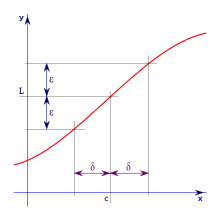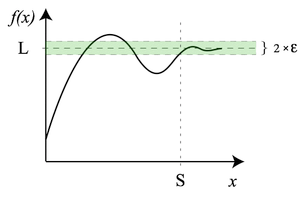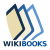# Limit (mathematics)

This is an overview of the idea of a limit in mathematics. For specific uses of a limit, see Limit of a sequence and Limit of a function.

In mathematics, a limit is the value that a function or sequence "approaches" as the input or index approaches some value. Limits are essential to calculus (and mathematical analysis in general) and are used to define continuity, derivatives, and integrals.

The concept of a limit of a sequence is further generalized to the concept of a limit of a topological net, and is closely related to limit and direct limit in category theory.

In formulas, a limit is usually written asand is read as "the limit of f of n as n approaches c equals L". Here "lim" indicates limit, and the fact that function f(n) approaches the limit L as n approaches c is represented by the right arrow (→), as in## Limit of a function

Main article: Limit of a functionWhenever a point x is within δ units of c, f(x) is within ε units of L.For all x > S, f(x) is within ε of L.

Suppose f is a real-valued function and c is a real number. Intuitively speaking, the expressionmeans that f(x) can be made to be as close to L as desired by making x sufficiently close to c. In that case, the above equation can be read as "the limit of f of x, as x approaches c, is L".

Augustin-Louis Cauchy in 1821, followed by Karl Weierstrass, formalized the definition of the limit of a function which became known as the (ε, δ)-definition of limit. The definition uses ε (the lowercase Greek letter epsilon) to represent any small positive number, so that "f(x) becomes arbitrarily close to L" means that f(x) eventually lies in the interval (L − ε, L + ε), which can also be written using the absolute value sign as |f(x) − L| < ε. The phrase "as x approaches c" then indicates that we refer to values of x whose distance from c is less than some positive number δ (the lower case Greek letter delta)—that is, values of x within either (c − δ, c) or (c, c + δ), which can be expressed with 0 < |xc| < δ. The first inequality means that the distance between x and c is greater than 0 and that xc, while the second indicates that x is within distance δ of c.

Note that the above definition of a limit is true even if f(c) ≠ L. Indeed, the function f need not even be defined at c.

For example, ifthen f(1) is not defined (see division by zero), yet as x moves arbitrarily close to 1, f(x) correspondingly approaches 2:

 f(0.9) f(0.99) f(0.999) f(1.0) f(1.001) f(1.01) f(1.1) 1.900 1.990 1.999 ⇒ undefined ⇐ 2.001 2.010 2.100

Thus, f(x) can be made arbitrarily close to the limit of 2 just by making x sufficiently close to 1.

In other words,This can also be calculated algebraically, asfor all real numbers x ≠ 1.

Now since x + 1 is continuous in x at 1, we can now plug in 1 for x, thus.

In addition to limits at finite values, functions can also have limits at infinity. For example, consider• f(100) = 1.9900
• f(1000) = 1.9990
• f(10000) = 1.99990

As x becomes extremely large, the value of f(x) approaches 2, and the value of f(x) can be made as close to 2 as one could wish just by picking x sufficiently large. In this case, the limit of f(x) as x approaches infinity is 2. In mathematical notation,## Limit of a sequence

Main article: Limit of a sequence

Consider the following sequence: 1.79, 1.799, 1.7999,... It can be observed that the numbers are "approaching" 1.8, the limit of the sequence.

Formally, suppose a1, a2, ... is a sequence of real numbers. It can be stated that the real number L is the limit of this sequence, namely:"The limit of an as n approaches infinity equals L"

to mean

For every real number ε > 0, there exists a natural number N such that for all n > N, we have |anL| < ε.

Intuitively, this means that eventually all elements of the sequence get arbitrarily close to the limit, since the absolute value |anL| is the distance between an and L. Not every sequence has a limit; if it does, it is called convergent, and if it does not, it is divergent. One can show that a convergent sequence has only one limit.

The limit of a sequence and the limit of a function are closely related. On one hand, the limit as n goes to infinity of a sequence a(n) is simply the limit at infinity of a function defined on the natural numbers n. On the other hand, a limit L of a function f(x) as x goes to infinity, if it exists, is the same as the limit of any arbitrary sequence an that approaches L, and where an is never equal to L. Note that one such sequence would be L + 1/n.

## Limit as "standard part"

In non-standard analysis (which involves a hyperreal enlargement of the number system), the limit of a sequencecan be expressed as the standard part of the valueof the natural extension of the sequence at an infinite hypernatural index n=H. Thus,.

Here the standard part function "st" rounds off each finite hyperreal number to the nearest real number (the difference between them is infinitesimal). This formalizes the natural intuition that for "very large" values of the index, the terms in the sequence are "very close" to the limit value of the sequence. Conversely, the standard part of a hyperrealrepresented in the ultrapower construction by a Cauchy sequence, is simply the limit of that sequence:.

In this sense, taking the limit and taking the standard part are equivalent procedures.

## Convergence and fixed point

A formal definition of convergence can be stated as follows. Supposeasgoes fromtois a sequence that converges to, withfor all. If positive constantsandexist withthenasgoes fromtoconverges toof order, with asymptotic error constantGiven a functionwith a fixed point, there is a nice checklist for checking the convergence of the sequence.

1) First check that p is indeed a fixed point:2) Check for linear convergence. Start by finding. If....then there is linear convergenceseries divergesthen there is at least linear convergence and maybe something better, the expression should be checked for quadratic convergence
3) If it is found that there is something better than linear the expression should be checked for quadratic convergence. Start by findingIf....then there is quadratic convergence provided thatis continuousthen there is something even better than quadratic convergencedoes not exist then there is convergence that is better than linear but still not quadratic



## Topological net

Main article: Net (topology)

All of the above notions of limit can be unified and generalized to arbitrary topological spaces by introducing topological nets and defining their limits.

An alternative is the concept of limit for filters on topological spaces.The Wikibook Calculus has a page on the topic of: Limits